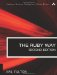# Section 2.25. Encoding and Decoding rot13 Text

### 2.24. Converting Strings to Numbers (Decimal and Otherwise)

Basically there are two ways to convert strings to numbers: the Kernel method Integer and Float and the to_i and to_f methods of String. (Capitalized method names such as Integer are usually reserved for special data conversion functions like this.)

The simple case is trivial, and these are equivalent:

`x = "123".to_i          # 123 y = Integer("123")      # 123`

When a string is not a valid number, however, their behaviors differ:

`x = "junk".to_i         # silently returns 0 y = Integer("junk")     # error`

to_i stops converting when it reaches a non-numeric character, but Integer raises an error:

`x = "123junk".to_i      # 123 y = Integer("123junk")  # error`

Both allow leading and trailing whitespace:

`x = " 123 ".to_i        # 123 y = Integer(" 123 ")    # 123`

Floating point conversion works much the same way:

`x = "3.1416".to_f      # 3.1416 y = Float("2.718")     # 2.718`

Both conversion methods honor scientific notation:

`x = Float("6.02e23")   # 6.02e23 y = "2.9979246e5".to_f # 299792.46`

to_i and Integer also differ in how they handle different bases. The default, of course, is decimal or base ten; but we can work in other bases also. (The same is not true for floating point.)

When talking about converting between numeric bases, strings always are involved. After all, an integer is an integer, and they are all stored in binary.

Base conversion, therefore, always means converting to or from some kind of string. Here we're looking at converting from a string. (For the reverse, see section 5.18 "Performing Base Conversions" and section 5.5 "Formatting Numbers for Output.")

When a number appears in program text as a literal numeric constant, it may have a "tag" in front of it to indicate base. These tags are 0b for binary, a simple 0 for octal, and 0x for hexadecimal.

These tags are honored by the Integer method but not by the to_i method:

`x = Integer("0b111")     # binary      - returns 7 y = Integer("0111")      # octal       - returns 73 z = Integer("0x111")     # hexadecimal - returns 291 x = "0b111".to_i         # 0 y = "0111".to_i          # 0 z = "0x111".to_i          # 0`

to_i, however, allows an optional second parameter to indicate base. Typically, the only meaningful values are 2, 8, 10 (the default), and 16. However, tags are not recognized even with the base parameter.

`x = "111".to_i(2)        # 7 y = "111".to_i(8)        # octal       - returns 73 z = "111".to_i(16)       # hexadecimal - returns 291 x = "0b111".to_i         # 0 y = "0111".to_i          # 0 z = "0x111".to_i         # 0`

Because of the "standard" behavior of these methods, a digit that is inappropriate for the given base will be treated differently:

`x = "12389".to_i(8)      # 123    (8 is ignored) y = Integer("012389")    # error  (8 is illegal)`

Although it might be of limited usefulness, to_i handles bases up to 36, using all letters of the alphabet. (This may remind you of the base64 encoding; for information on that, see section 2.37, "Encoding and Decoding base64 Strings.")

`x = "123".to_i(5)        # 66 y = "ruby".to_i(36)      # 1299022`

It's also possible to use the scanf standard library to convert character strings to numbers. This library adds a scanf method to Kernel, to IO, and to String:

`str = "234 234 234" x, y, z = str.scanf("%d %o %x")    # 234, 156, 564`

The scanf methods implement all the meaningful functionality of their C counterparts scanf, sscanf, and fscanf. It does not handle binary.The Ruby Way, Second Edition: Solutions and Techniques in Ruby Programming (2nd Edition)
ISBN: 0672328844
EAN: 2147483647
Year: 2004
Pages: 269
Authors: Hal Fulton

Similar book on Amazon